General Results of the Theory of Relativity

Author: Albert Einstein

# General Results of the Theory of Relativity

## Albert Einstein

It is clear from our previous considerations that the (special) theory of relativity has grown out of electrodynamics and optics. In these fields it has not appreciably altered the predictions of theory, but it has considerably simplified the theoretical structure, i.e. the derivation of laws, and—what is incomparably more important—it has considerably reduced the number of independent hypotheses forming the basis of theory. The special theory of relativity has rendered the Maxwell-Lorentz theory so plausible, that the latter would have been generally accepted by physicists even if experiment had decided less unequivocally in its favour.

Classical mechanics required to be modified before it could come into line with the demands of the special theory of relativity. For the main part, however, this modification affects only the laws for rapid motions, in which the velocities of matter v are not very small as compared with the velocity of light. We have experience of such rapid motions only in the case of electrons and ions; for other motions the variations from the laws of classical mechanics are too small to make themselves evident in practice. We shall not consider the motion of stars until we come to speak of the general theory of relativity. In accordance with the theory of relativity the kinetic energy of a material point of mass m is no longer given by the well-known expression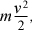but by the expression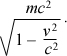This expression approaches infinity as the velocity v approaches the velocity of light c. The velocity must therefore always remain less than c, however great may be the energies used to produce the acceleration. If we develop the expression for the kinetic energy in the form of a series, we obtain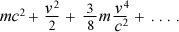When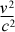is small compared with unity, the third of these terms is always small in comparison with the second, which last is alone considered in classical mechanics. The first term mc2 does not contain the velocity, and requires no consideration if we are only dealing with the question as to how the energy of a point-mass depends on the velocity. We shall speak of its essential significance later.

The most important result of a general character to which the special theory of relativity has led is concerned with the conception of mass. Before the advent of relativity, physics recognised two conservation laws of fundamental importance, namely, the law of the conservation of energy and the law of the conservation of mass; these two fundamental laws appeared to be quite independent of each other. By means of the theory of relativity they have been united into one law. We shall now briefly consider how this unification came about, and what meaning is to be attached to it.

The principle of relativity requires that the law of the conservation of energy should hold not only with reference to a co-ordinate system K, but also with respect to every co-ordinate system K’ which is in a state of uniform motion of translation relative to K, or, briefly, relative to every "Galileian" system of co-ordinates. In contrast to classical mechanics, the Lorentz transformation is the deciding factor in the transition from one such system to another.

By means of comparatively simple considerations we are led to draw the following conclusion from these premises, in conjunction with the fundamental equations of the electrodynamics of Maxwell: A body moving with the velocity v, which absorbs an amount of energy E0 in the form of radiation without suffering an alteration in velocity in the process, has, as a consequence, its energy increased by an amount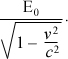In consideration of the expression given above for the kinetic energy of the body, the required energy of the body comes out to be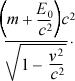Thus the body has the same energy as a body of mass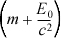moving with the velocity v. Hence we can say: If a body takes up an amount of energy E0, then its inertial mass increases by an amount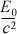; the inertial mass of a body is not a constant, but varies according to the change in the energy of the body. The inertial mass of a system of bodies can even be regarded as a measure of its energy. The law of the conservation of the mass of a system becomes identical with the law of the conservation of energy, and is only valid provided that the system neither takes up nor sends out energy. Writing the expression for the energy in the form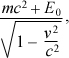we see that the term mc2, which has hitherto attracted our attention, is nothing else than the energy possessed by the body before it absorbed the energy E0.

A direct comparison of this relation with experiment is not possible at the present time, owing to the fact that the changes in energy E0 to which we can subject a system are not large enough to make themselves perceptible as a change in the inertial mass of the system.is too small in comparison with the mass m, which was present before the alteration of the energy. It is owing to this circumstance that classical mechanics was able to establish successfully the conservation of mass as a law of independent validity.

Let me add a final remark of a fundamental nature. The success of the Faraday-Maxwell interpretation of electromagnetic action at a distance resulted in physicists becoming convinced that there are no such things as instantaneous actions at a distance (not involving an intermediary medium) of the type of Newton’s law of gravitation. According to the theory of relativity, action at a distance with the velocity of light always takes the place of instantaneous action at a distance or of action at a distance with an infinite velocity of transmission. This is connected with the fact that the velocity c plays a fundamental rle in this theory. In Part II we shall see in what way this result becomes modified in the general theory of relativity.

### Related Resources

Albert Einstein

Title: General Results of the Theory of Relativity

Select an option:

## Email Options

Title: General Results of the Theory of Relativity

Select an option: